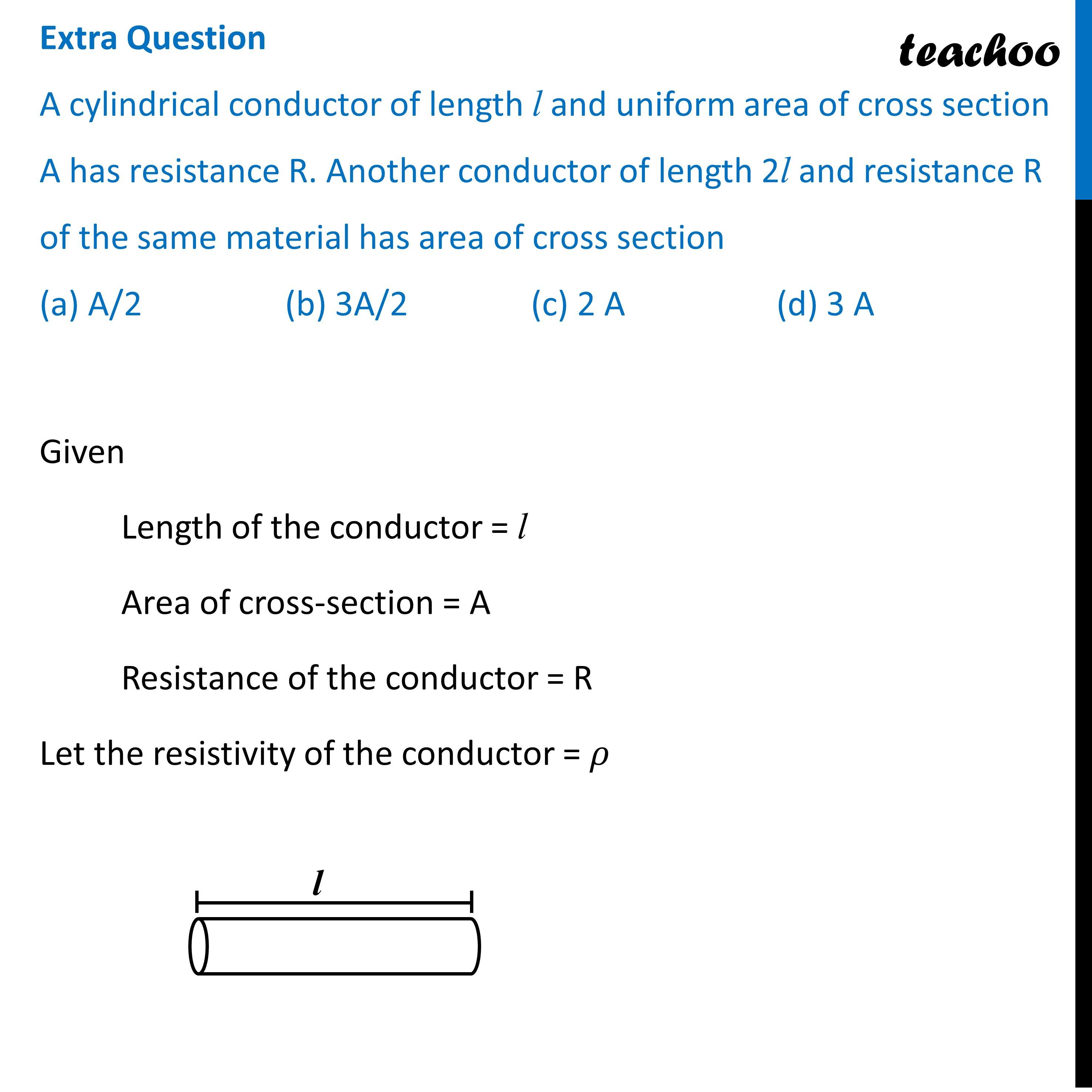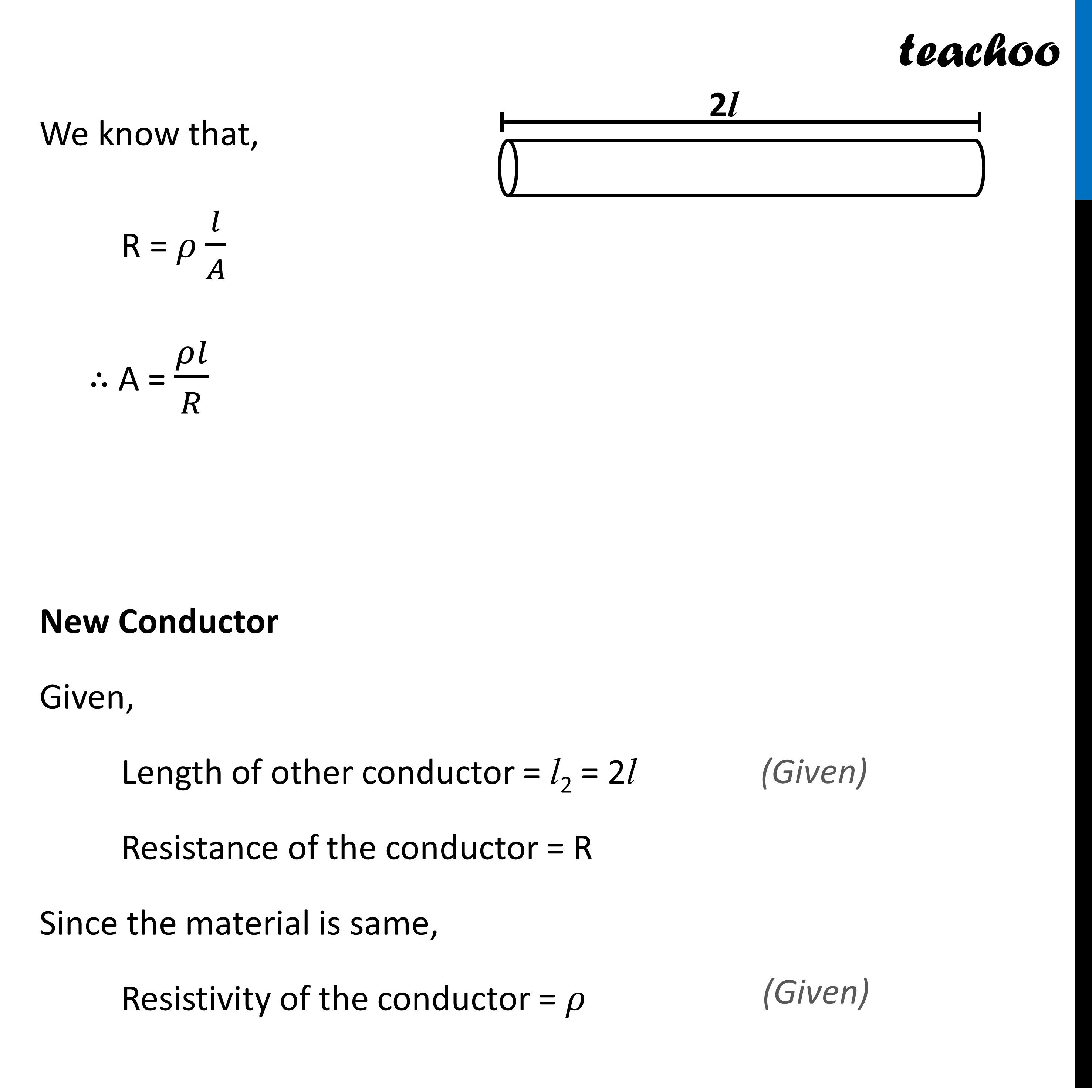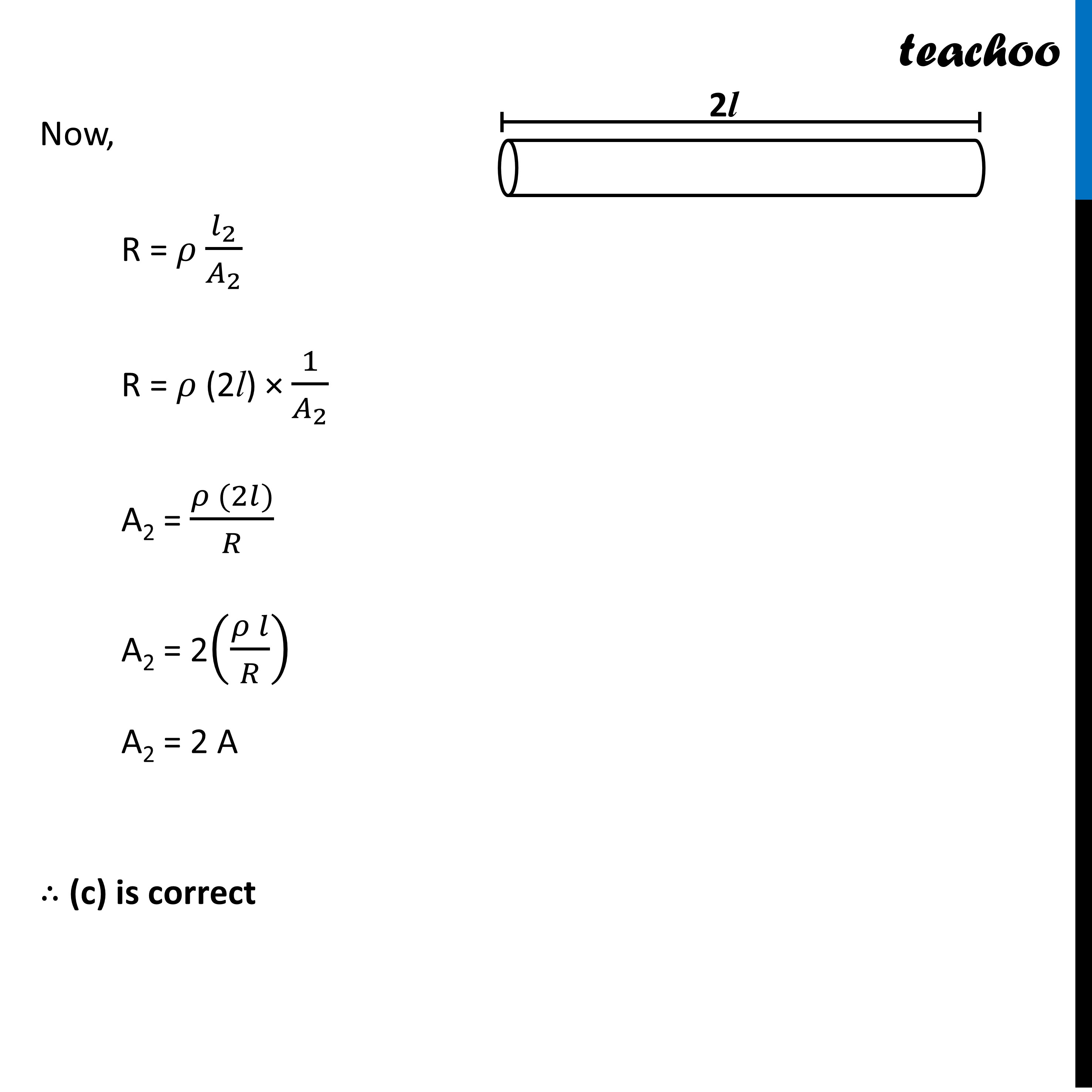MCQs from (Past Year Papers & NCERT Exemplar)

Class 10
Chapter 12 Class 10 - Electricity

## (d) 3 ALearn in your speed, with individual attention - Teachoo Maths 1-on-1 Class

### Transcript

Extra Question A cylindrical conductor of length l and uniform area of cross section A has resistance R. Another conductor of length 2l and resistance R of the same material has area of cross section (a) A/2 (b) 3A/2 (c) 2 A (d) 3 AGiven Length of the conductor = l Area of cross-section = A Resistance of the conductor = R Let the resistivity of the conductor = 𝜌 We know that, R = 𝜌 𝑙/𝐴 ∴ A = 𝜌𝑙/𝑅 New Conductor Given, Length of other conductor = l2 = 2l Resistance of the conductor = R Since the material is same, Resistivity of the conductor = 𝜌 Now, R = 𝜌 𝑙_2/𝐴_2 R = 𝜌 (2l) × 1/𝐴_2 A2 = (𝜌 (2𝑙))/𝑅 A2 = 2((𝜌 𝑙)/𝑅) A2 = 2 A ∴ (c) is correct